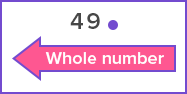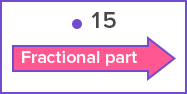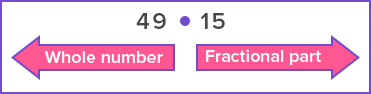# Decimal Point - Definition with Examples

The Complete K-5 Math Learning Program Built for Your Child

• 30 Million Kids

Loved by kids and parent worldwide

• 50,000 Schools

Trusted by teachers across schools

• Comprehensive Curriculum

Aligned to Common Core

What is Decimal Point?

It is a point or dot we use to separate the whole number part from the fractional part of a decimal number.

A decimal number is a number that consists of a whole number and a fractional part. The decimal point separates the whole number from the fractional part.

Example:In the number 258.16, the decimal point separates the whole number 258 from the fractional part 16, which is 16 hundredths.

The decimal point is placed in between the ones and the tenths.The whole number is written to the left of the decimal point.The fractional part is written to the right of the decimal point.So, a decimal number looks like:The decimal point makes it easy to read a decimal number. We can read a decimal number  in two ways:

1. Using “and” in place of the decimal point:

In this method:

• The whole number part is read as is.

• The decimal point is read as “and”.

• Then the fractional part is read as is.

For example, the number 258.16 is read as “two hundred fifty-eight and sixteen hundredths”.2. Reading the decimal point as “point”:

In this method:

• The whole number part is read as is.

• The decimal point is read as “point”.

• Then the fractional part is read as individual digits.

For example, the number 258.16 is read as “two hundred fifty-eight point one six”.Fun facts The fractional part to the right of the decimal point denotes a number less than 1.   A whole number can also be represented as a decimal number by simply writing point zero ( .0) after the ones digit. For example, we can write 15 can as 15.0 The decimal point is also called as a decimal separator.

Won Numerous Awards & Honors